# Autoencoders and latent space fragmentation – X – a method to create suitable latent vectors for the generation of human face images

My present post series explores options to use a standard convolutional Autoencoder [AE] for the creation of images with human faces. The face generation should based on random input to the AE’s Decoder. On our quest for a suitable method we have meanwhile learned a lot about other aspects of Autoencoders, vector distributions in multi-dimensional latent spaces and generative methods for our special case:

• Methods to create statistical latent vectors [z-vectors] as input for the AE’s Decoder must be chosen carefully. Among other things: It is difficult to create a bunch of random vectors which cover wider areas in the vastness of a multidimensional space. So the z-vector creation must be adjusted to specific requirements.
• After having been trained with CelebA images a convolutional AE fills a limited and coherent region in the latent space with z-points for the training images. This latent space region appears to be critical for successful image creation: Statistically generated z-vectors should point to this region. The core of the z-point distribution gets filled relatively densely.
• A convolutional AE maps human face images onto an approximate multivariate normal distribution. This gives the inner core of the z-point distribution the structure of a multidimensional ellipsoid. The projections of this ellipsoid onto 2-dimensional coordinate planes show characteristic nested elliptic contour lines.
• As the main axes of these ellipses were inclined with different angle towards the axes of chosen coordinate planes we concluded that linear correlations mark average dependencies between the z-vector components. Limiting conditions imposed by these correlations must also be fulfilled by z-vectors used as the Decoder’s input.

See previous posts in this series for more details. In particular, the last 2 posts

Autoencoders and latent space fragmentation – IX – PCA transformation of the z-point distribution for CelebA

Autoencoders and latent space fragmentation – VIII – approximation of the latent vector distribution by a multivariate normal distribution and ellipses

have shown that the density distribution for the z-points really exhibits elliptic contour lines in the original coordinate system of the latent space and (!) in the target coordinate system of a PCA transformation.

In this post we use our gathered knowledge: I present a first simple method to generate z-vectors which point to the latent space region filled by z-points for CelebA images. These z-vectors will fulfill the general and limiting elliptic conditions for their components.

# Decomposing the full problem of latent vector generation into a sequence of 2-dimensional problems

The nice thing about multivariate Gaussian distributions with linear correlations between the vector components is the following: We can reduce the problem of choosing proper component values to a series of 2-dimensional restrictions. Firstly we can use characteristic properties of the Gaussian distribution for each component. And secondly we can use confidence ellipses in 2-dimensional coordinate planes to restrict the component values to allowed intervals.

Ellipses are most easy to handle when their axes are aligned with the axes of the coordinate system in which we describe them. So, let us assume that we know an affine transformation T to a new coordinate system which also has orthogonal axes and supports the following special transformation properties for a multivariate normal density distribution:

1. T maps nested elliptic contour lines of the multidimensional density distribution and in particular confidence ellipses for component pairs in the original coordinate system to nested elliptic contours and confidence ellipses in the new coordinate system.
2. Taligns the centers of the transformed ellipses with the origin of the new coordinate system.
3. T aligns the main axes of the mapped ellipses with the axes of the new coordinate system.
4. T is reversible.

How could we then use the transformed data for vector-creation?

In the new coordinate system, a contour ellipse in a chosen coordinate plane for the axes-indices (i, j) may have main diameters of size

d1 = 2 * a    and    d2 = 2 * b.

We then can first select a random v_i value to fall into a range [-a * fact, a * fact].

fact * a    <    v_i    <    fact * a

With fact being a proper factor. This factor defines a confidence level in the new coordinate system. With the value of v_i fixed and b being the half-diameter in the orthogonal direction the correlation condition for the z-point distribution says that the v_j value must fall into an interval [-c, c] defined by:

-c    <    v_j    <    c,
with c = b * fact * sqrt(1 – x**2 / (fact * a)**2)

But within these limits we can again choose the v_j-value freely. Below I use a simple random-function for a constant probability density to pick a value.

However: It would not be enough to restrict the coordinates to the conditions of just one ellipse! The components of the created vectors must in parallel fulfill elliptic conditions for all of the possible pairs of vector-components. I.e. we may need to adapt the v_j values gained from the analysis of a fist 2D-ellipse to further conditions of other ellipses and component pairs. This can be achieved by an iteration. For z_dim = 256 this involves a total of 32640 checks and possible value-adaptions to each and all of the allowed value ranges.

In addition: The order by which the component-pairs and their conditions are investigated must be randomized to get real statistical vector distributions.

Eventually the resulting vector components must be re-transformed into the original coordinate system of the latent space.

The ellipse for the “core’s boundary” in the original coordinate system will be defined by the chosen confidence level of the ellipsoidal normal distribution. We saw already that a confidence level of σ = 2.0 defines the transition to outer regions of the z-point density distribution quite well.

This all sounds manageable by relative simple Python programs. But: Do we know a proper transformation T? Yes, we do: A PCA-transformation of the z-point density distribution has all the properties discussed above.

# Using half maximum values after a PCA transformation of the z-point distribution

The last post proved that a PCA transformation maps ellipses onto ellipses for component pairs in the transformed PCA coordinate system. The advantage of the ellipses there is that their main axes are on average well aligned with the orthogonal PCA coordinate axes. Gaussians for the number density distribution per component are mapped to Gaussians for the new components in the transformed coordinate system. So, the basic idea for a proper z-vector generation is:

1. Take the multivariate normal z-point distribution for the training images in the AE’s latent space.
2. Apply a PCA analysis to diagonalize the correlation matrix and transform the z-vector components to the PCA coordinate system.
3. Use the ellipses in coordinate planes of the PCA coordinate system to create random z-vector components fulfilling all required conditions there.
4. Re-transform the resulting z-vector components into the original coordinate system of the latent space.

Point 3 in our method is covered by a numerical analysis of the Gaussians in the PCA-coordinate system. We determine the half-width numerically by analyzing the density distribution with the help of sampling intervals. This simple method has resolution limits related to the size of the sampling interval. This has consequences for PCA components with a small standard deviation. We saw already in the last posts that such distributions appear for higher PCA components at the lower end of the explained variance.

# Does the suggested method work?

The convolutional AE we work with was defined in previous posts with 4 Conv2D layers in the Encoder and 4 Conv2DTranspose layers in Decoder. The number of latent space dimensions was z_dim = 256. The AE network was trained on CelebA images. I do not want to bore you with details of the codes for the creation of z-vectors consistent to the resulting elliptic conditions. It is all standard. The PCA-transformation can e.g. be taken from the sklearn-package.

I have applied a constant probability density to choose a random value within the allowed ranges for the component values of the aspired z-vectors in the PCA coordinate system. For the plots below I have used the most important 50 to 105 PCA components (out of 256). The plots include confidence ellipses on a level of σ = 2.2. I derived the confidence ellipses by directly evaluating the standard deviations of the transformed distribution data in all coordinate directions.

The first plot shows you such an ellipse for the coordinate plane corresponding to the first two, most important PCA components. The orange points mark 20 z-points defined by 20 randomly z-vectors fulfilling all elliptic conditions. The plot contains 120,000 z-points for images out of the 170,000 CelebA pictures used during training.

Generated statistical vectors in the PCA coordinate system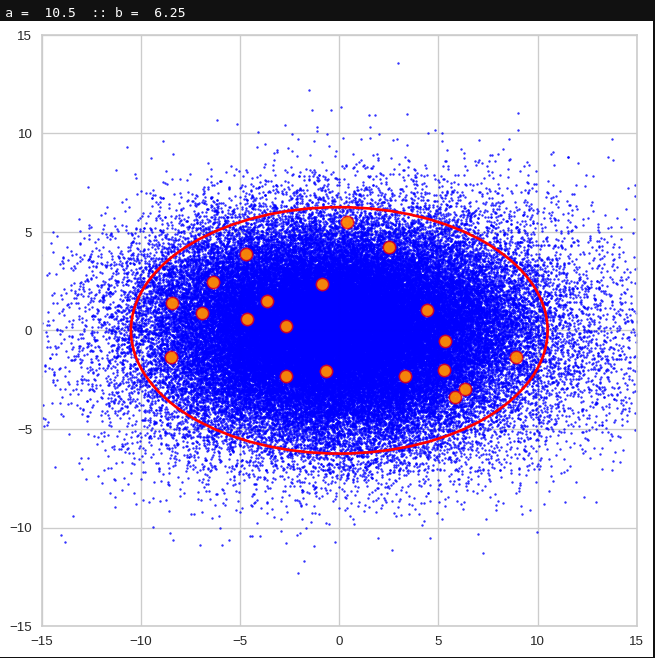For elliptic contour lines see the last post before the present one in this series. The next plot shows the same generated 20 z-vectors for other component-combinations among the first 20 of the most important PCA-components. The plots contain a selection of 60,000 z-points.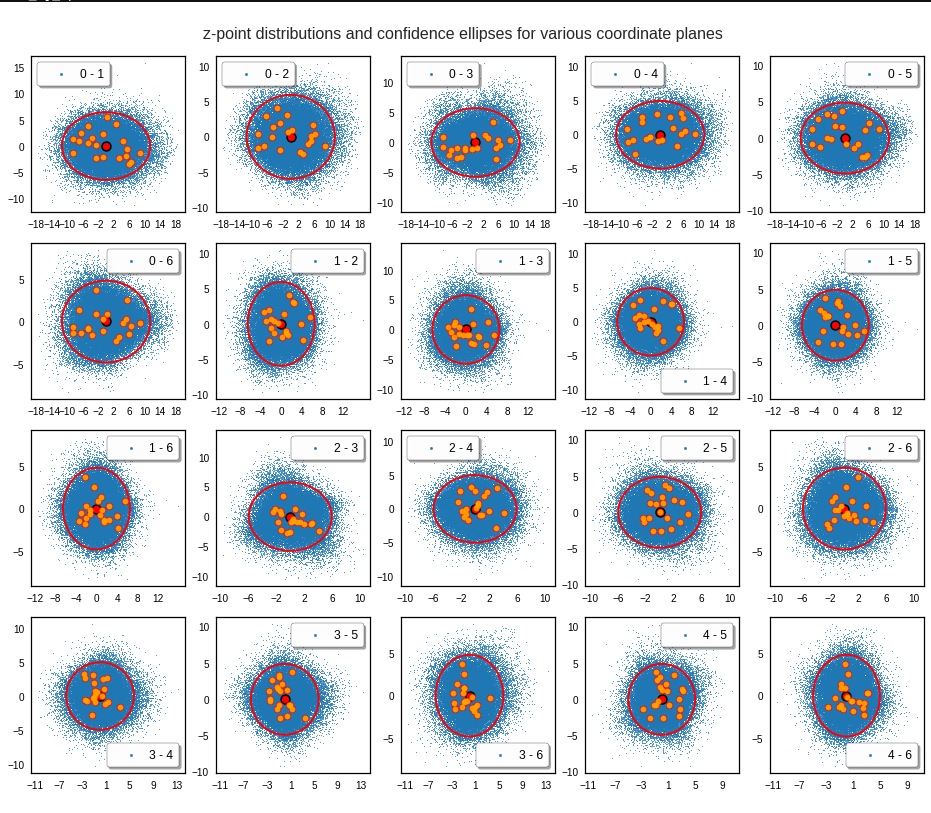The outer z-points points do not always indicate that we have elliptic contours in the denser core of the displayed 2-dimensional distributions. But see the last post for proofs that the inner core inside the red ellipse really displays elliptic contours. You see that all random vectors lie within the 2-σ-ellipses.

The next plot shows the generated z-vectors in the original coordinate system of the latent space. The component values were back-transformed from the PCA-system to the original coordinate system.

Generated statistical z-vectors after an inverse PCA transformation to the original coordinate system of the latent space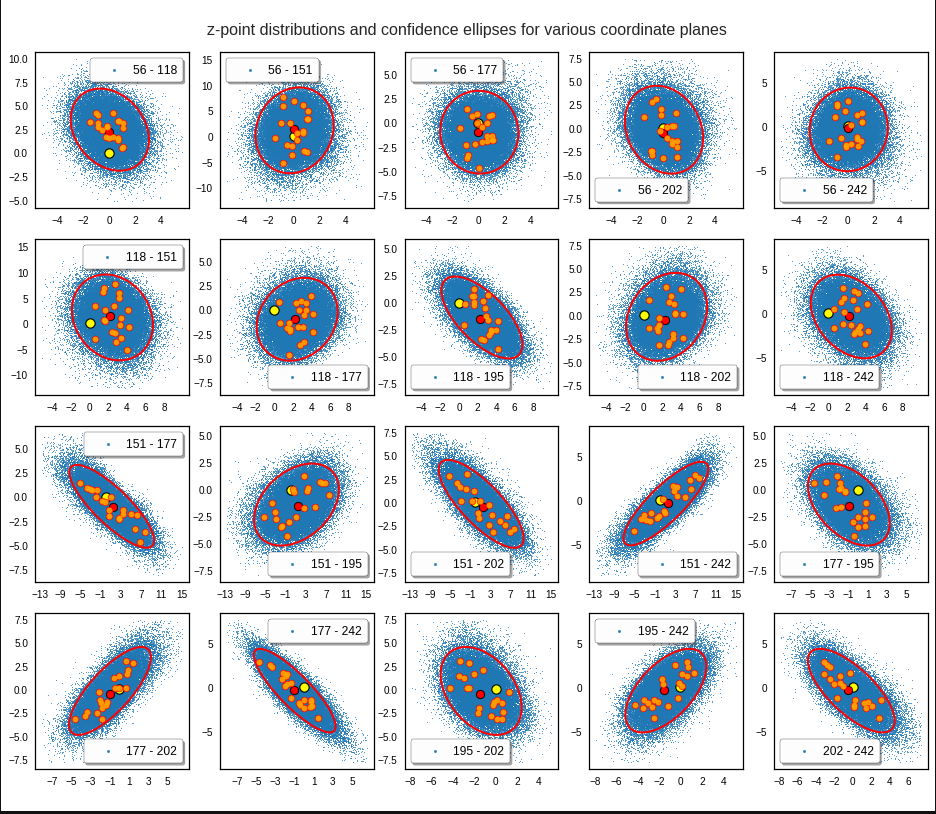We get similar plots for other component pairs. And of course for other generated vectors.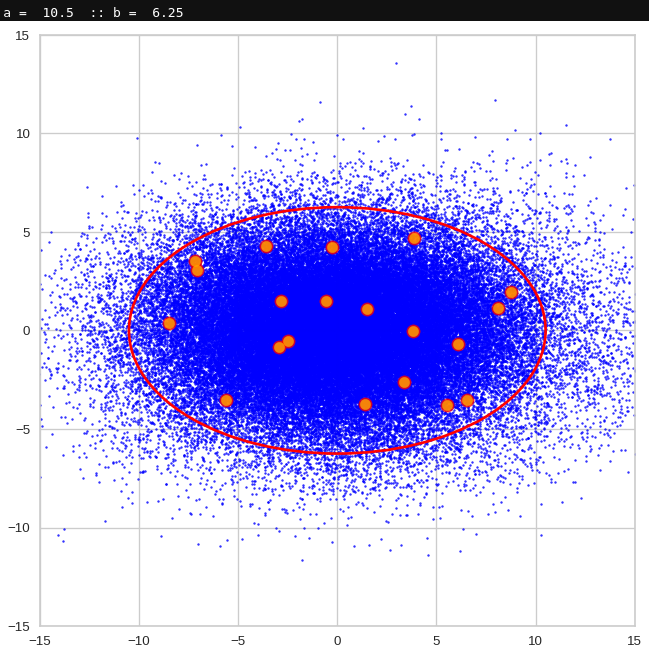Generated statistical z-vectors in the PCA coordinate system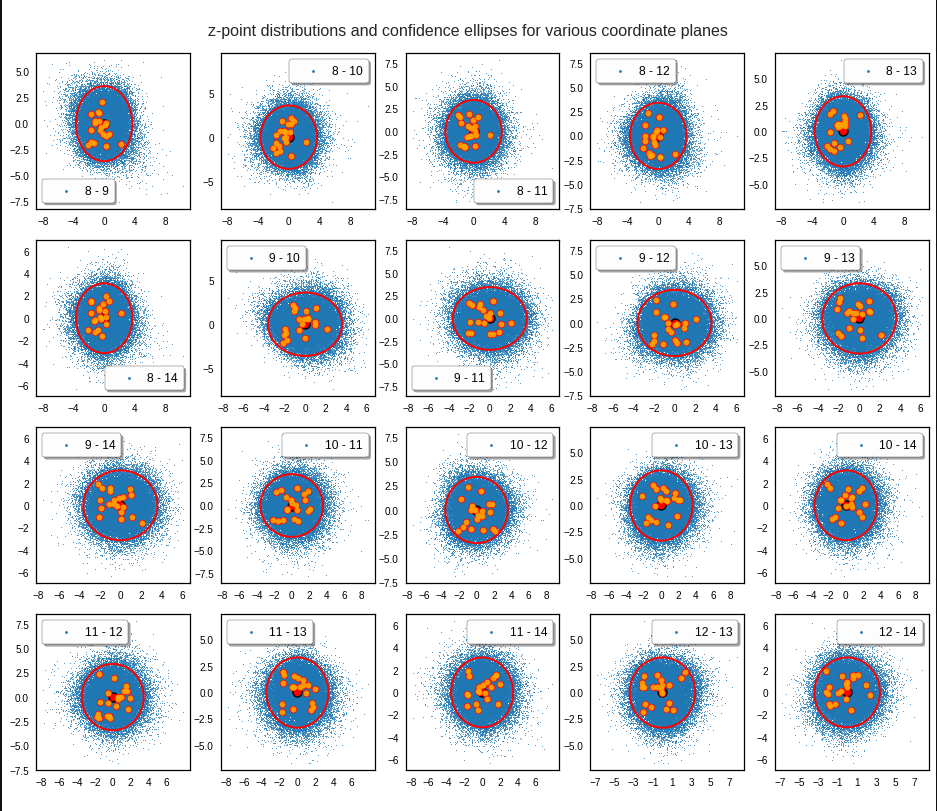Generated statistical z-vectors after an inverse PCA transformation to the original coordinate system of the latent space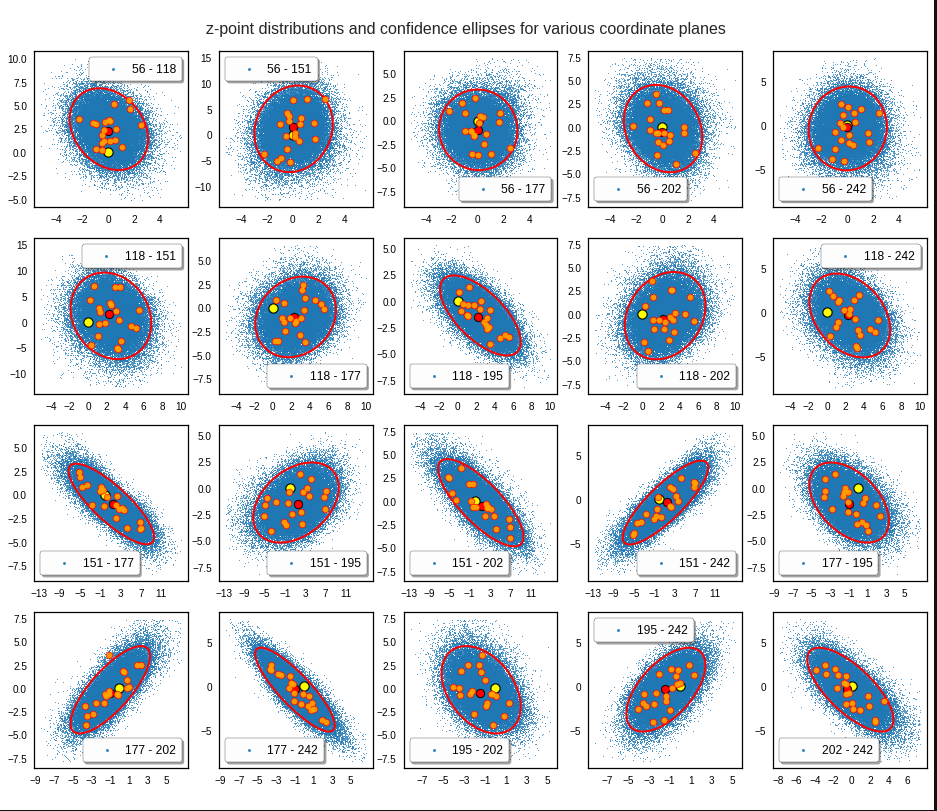Technically we have obviously achieved what we wanted: Our generated statistical vectors are distributed within the core of our multidimensional ellipsoid.

Note that this method fortunately works even when we use a limited number of the PCA components, only. This is due to intricate properties of a PCA transformation which guarantee that a back-transformation puts the resulting points close to the original ones even when we omit less important PCA components. I cannot discuss the math-details in this blog. You have to see scientific literature for this. An introduction is e.g. provided by https://arxiv.org/pdf/1404.1100.pdf.

For me this property of the PCA transformation was helpful when I ran into the resolution problem for a proper half-width of the Gaussians. Taking 256 components lead to errors as elliptic conditions for very narrow Gaussians were not properly defined and some of the created vectors left the allowed value ranges.

# Resulting face images

Let us look at some results. First I want to remind you from where we started:

Failed trials with improper random z-vectors based on constant probability densities

A simple random generator used in the beginning was totally inapt to feed the AE’s Decoder with proper statistical z-vectors. And now – look at the following plots. They were produced for a varying number of PCA components between 50 and 120, 100000 statistically selected z-points within a 3 σ-level for the PCA-transformation and various factors 0.6 < fact < 0.8 used upon a half-width corresponding to a confidence level of 2.35 σ: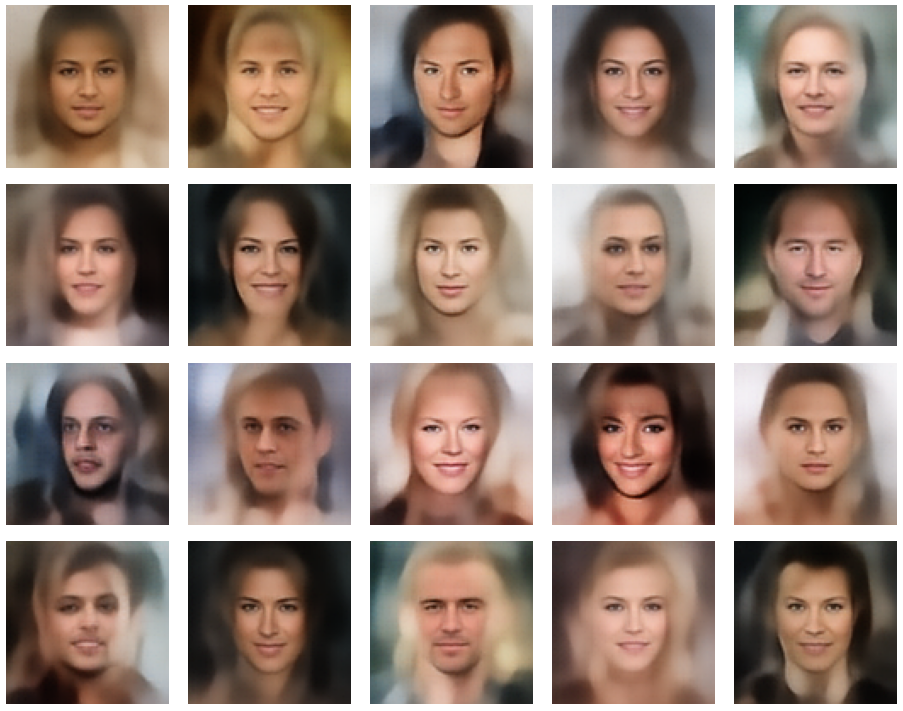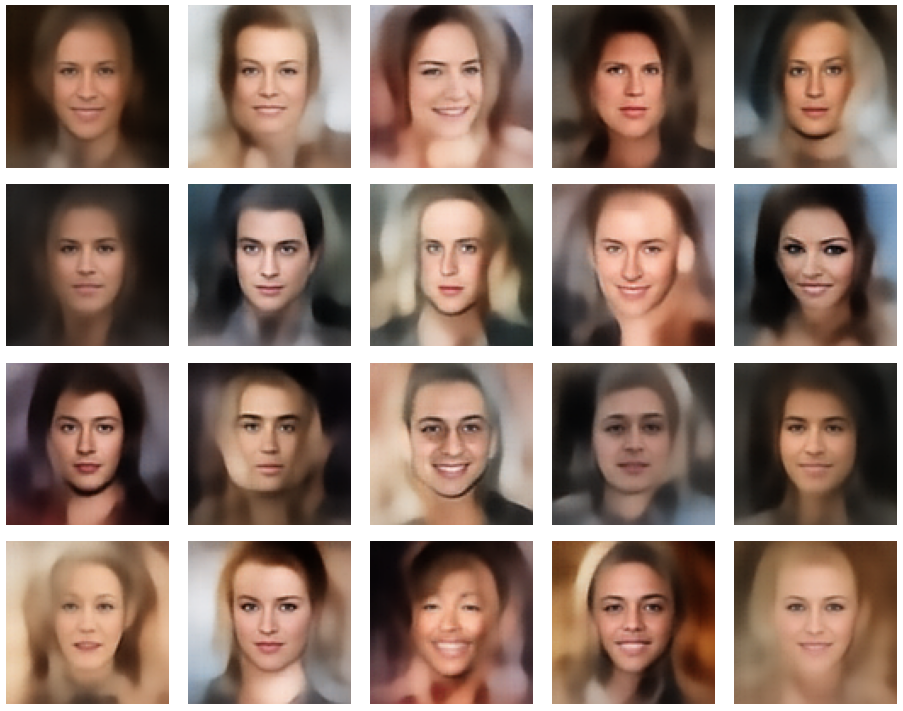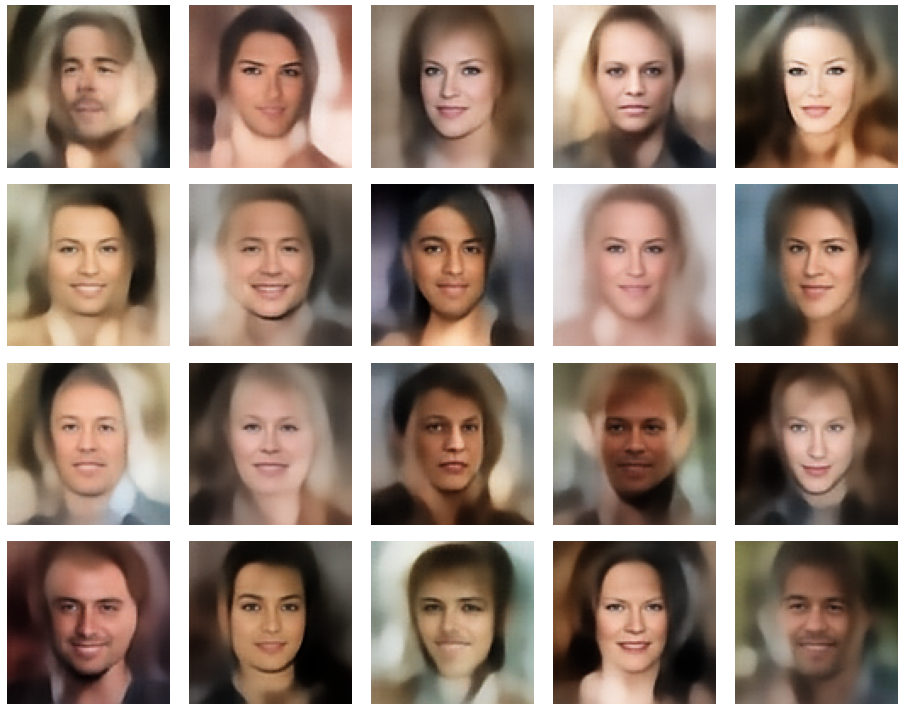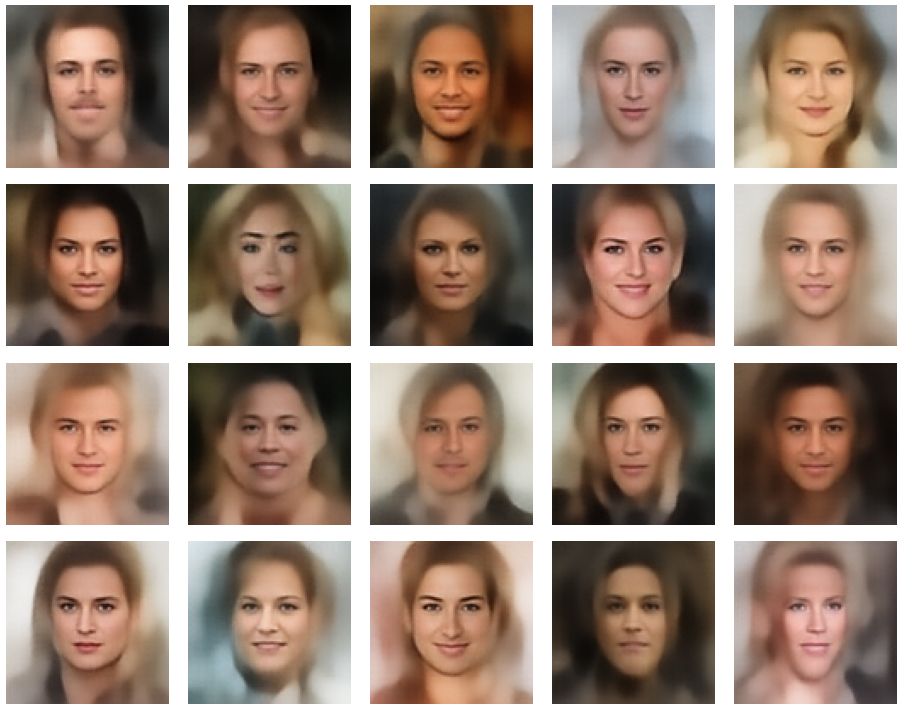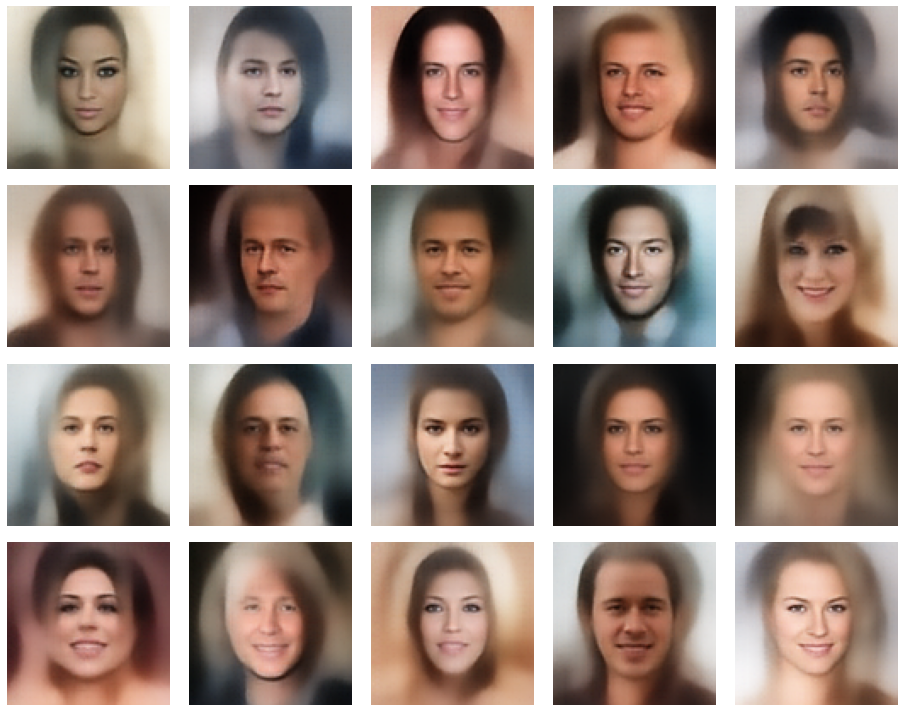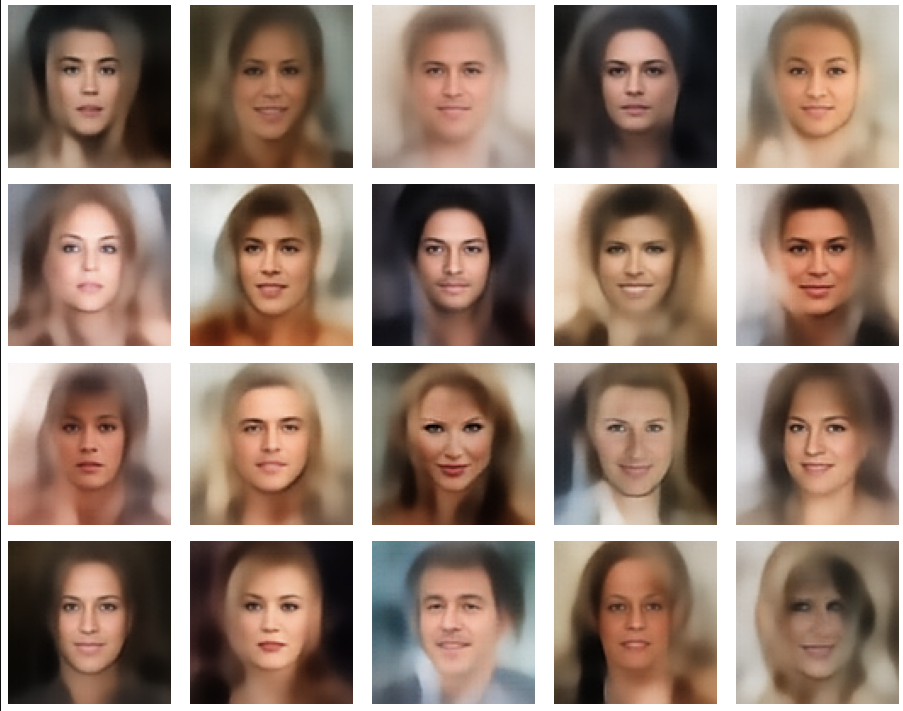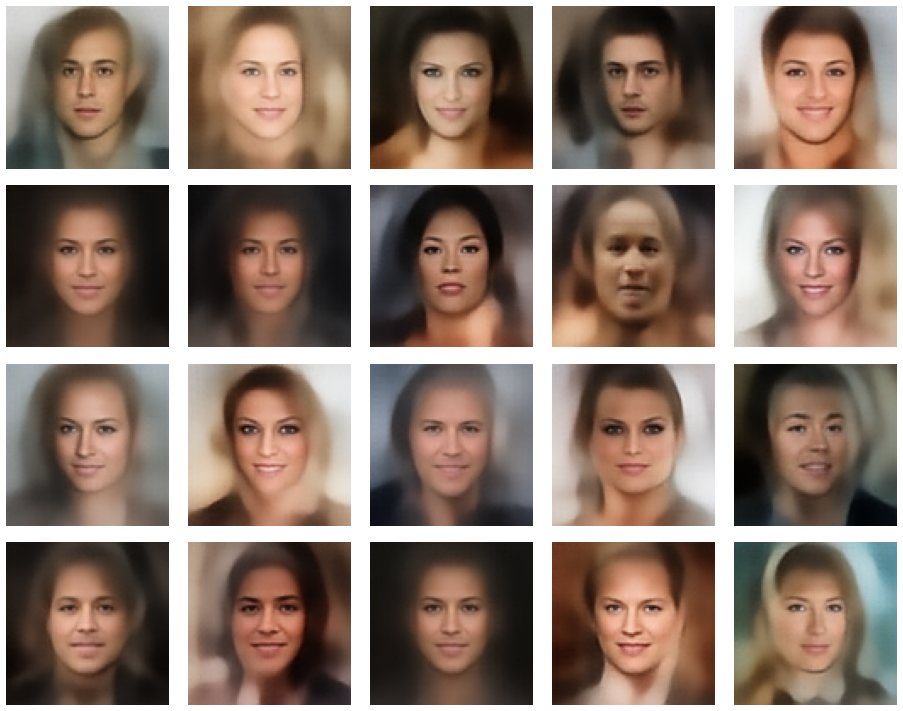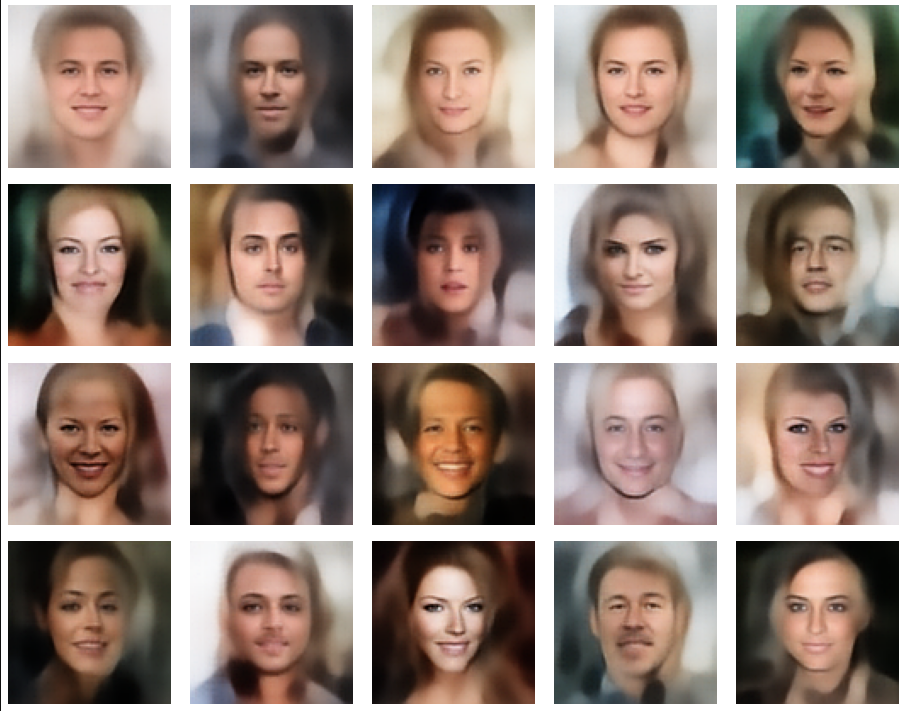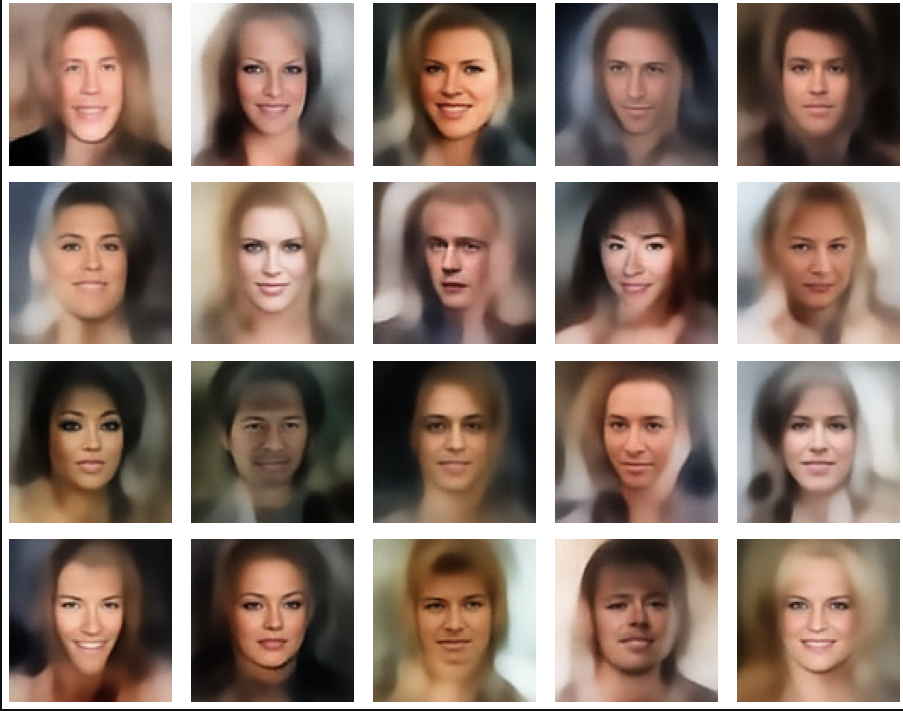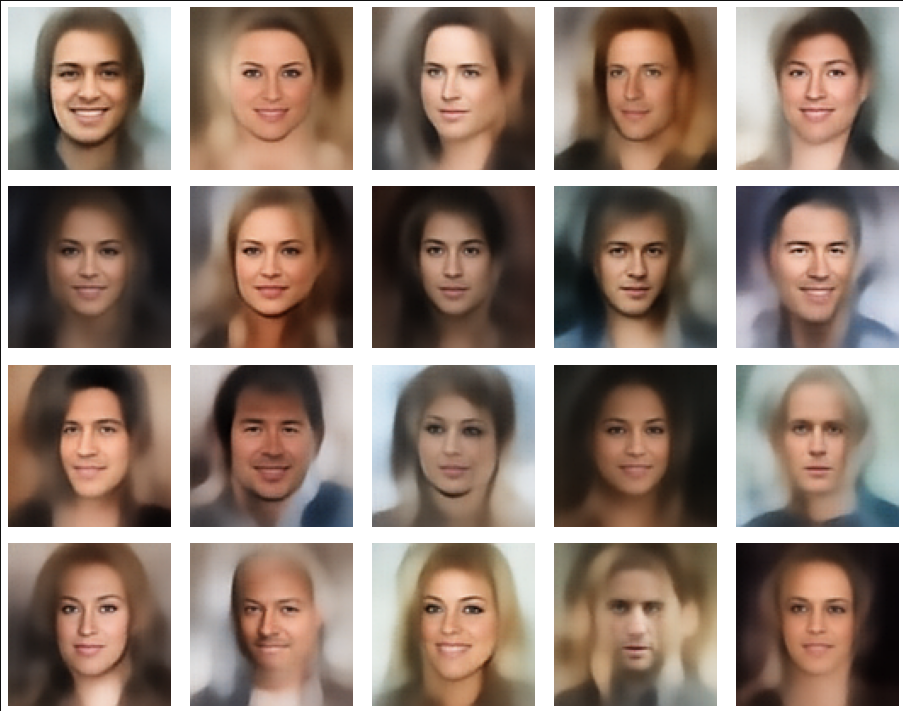In some cases – for a higher number of PCA components – we even see smaller details of the face images and a reasonable transition to some kind of hairdo. Please remember that z_dim = 256 is a pretty low number for the latent space to cover the encoding of face details. And celebrities as covered by CelebA use make-up ….

In case you think the above result is not noteworthy: Please remember that we talk about a simple standard Autoencoder and not about a Variational Autoencoder and neither about a transformer based Autoencoder. No fancy additions to cost functions or special layers. And who ever has read the very instructive book of D. Foster on “Generative Deep Learning” (1st edition, O’Reilly) may compare his images to mine. And I have used a lower resolution of the original images than D. Foster. Just to motivate people to look a bit deeper into properties of data distributions in latent spaces.

# Conclusion and outlook

We have come a lot closer to our objective of using a standard minimal Autoencoder for generative purposes. On our way, we got a much deeper understanding of the vector-distribution a trained AE creates in its latent space for human face images.

The method presented in this post to create reasonable statistical z-vectors still has its limits and there is a lot of open space for improvements. Attentive readers may e.g. ask: Why did he not use confidence ellipses directly? And why not the ellipses found in the original coordinate system of the latent space? And what about micro-correlations? And are there clusters for certain properties as the hair-color, sex, smiling, etc. in the multivariate z-point distribution in the AE’s latent space?

I will discuss these topics in further posts. In the meantime keep in mind that the basic point for turning a standard Autoencoder into a generative tool is to understand how it fills its latent space.

Note also that I myself have speculated in other posts of this blog that failures of using standard AEs for generative purposes may have their ultimate reason in the micro-structure of the z-point distribution. The present results render these previous ideas of mine plain wrong.

# Links to previous posts of this series

Autoencoders and latent space fragmentation – IX – PCA transformation of the z-point distribution for CelebA

Autoencoders and latent space fragmentation – VIII – approximation of the latent vector distribution by a multivariate normal distribution and ellipses

Autoencoders and latent space fragmentation – VII – face images from statistical z-points within the latent space region of CelebA

Autoencoders and latent space fragmentation – VI – image creation from z-points along paths in selected coordinate planes of the latent space

Autoencoders and latent space fragmentation – V – reconstruction of human face images from simple statistical z-point-distributions?

Autoencoders and latent space fragmentation – IV – CelebA and statistical vector distributions in the surroundings of the latent space origin

Autoencoders and latent space fragmentation – III – correlations of latent vector components

Autoencoders and latent space fragmentation – II – number distributions of latent vector components

Autoencoders and latent space fragmentation – I – Encoder, Decoder, latent space

And before we forget it: Besides the Putler in the east there is also an extremist right-wing, semi-fascistic party in Germany on a record high support level in the population of 18%. This is a party which wants to stop all sanctions against the Russian aggressor in the ongoing war in Ukraine. You see the pattern behind this? This party is presently becoming bigger in number of supporters than the government leading social democrats. So, there is more at stake at present in Europe than the war in Ukraine. We need to defend our democracies with all the means of democracies. And its time to ask for more decisive legal action against a party which already is under observation of the German internal secret service.

# Autoencoders and latent space fragmentation – II – number distributions of latent vector components

This post series studies the (in-) ability of a trained Autoencoder [AE] to create reasonable human face images from statistical vectors placed in its latent space. In my last post

Autoencoders and latent space fragmentation – I – Encoder, Decoder, latent space

I have described what the purposes of the two sub-networks of an AE, i.e. the Encoder and the Decoder, are. We saw that the so called latent space plays an important role for the interplay of these sub-networks: Vectors in the latent space – z-pointsencode properties of objects presented to the Autoencoder, more precisely to its Encoder. The bunch of objects used during training thus gives us a distribution of vectors and respective z-points within certain regions of the latent space. The Decoder reconstructs objects from latent vectors.

One of my eventual objectives in this series is the creation of new objects of the same class presented to the AE during its training. I focus on the special case of images displaying human faces. Therefore, I have trained a convolutional AE with the so called CelebA dataset. After training one may hope that an AE will be able to produce images with new faces, which are not present in CelebA, when we feed the Decoder with suitable z-points. The question is what “suitable z-points” are and where they are located in the latent space.

Of course, I want to use the Decoder’s reconstruction abilities to achieve my goal. To get new faces, a statistical element is a must. The basic idea is to use statistically created z-points as input for the Decoder.

# Objective of this post

In the first post of this series I have already indicated that not all vectors in the AE’s latent space may lead to the production of reasonable images. It might well be that we must hit certain confined regions of the latent space. An interesting question, therefore, is the following:

Are all generators of statistical vectors suitable to hit the regions of a latent space which an Autoencoder will fill for certain training objects?

In this and further posts I want to show you that this is not the case. A bad choice of a statistical generating method in combination with the high number of latent space dimensions may lead to a complete failure.

To achieve this insight we must study both the real z-point distribution which an AE creates for CelebA images and the artificial vector or z-point distribution coming from a specific statistical generator. In this post I study a generator which assigns the vector components values which are taken from a real number interval with a constant probability. The number of dimensions N of the latent space shall be N=256.

We shall see that we indeed get confronted with a special side of the curse of high dimensionality and that our artificial z-point distribution does not match the real one for CelebA at all if we do not restrict parameters in a somewhat counter-intuitive way. As a side effect we will learn that our AE organizes the latent vector distributions for CelebA images via functions very similar to Gaussians. Furthermore, we shall see that we speak of just one coherent and confined z-point region with very small extensions. The center of this region sits close to a hypervolume spanned by only a few (out of 256) coordinate axes.

# Methods to create statistical z-points

To create statistical z-points in a latent space we have to employ some generating mechanism for respective statistical vectors. See my first post for the correspondence of z-points to latent vectors. There are multiple ways available to create latent vectors. I just name 3 popular ones:

1. Create a dense homogeneous distribution by filling some volume around the origin with a grid of points.
2. Use a constant probability density for values in a real number interval [-b, b], pick such values statistically and assign them to individual vector components.
3. Use one or multiple Gaussian distributions to define the vector component values.

Note that when applying the second and the third method the statistics works on the level of the vector components. These components are handled as independent variables, each obeying a certain probability distribution of assignable real values.

A small calculation shows that method 1 will not work in practice, as such a distribution requires an enormous number of points for high dimensions and a decent resolution. Method 2 looks simple and works well in 2D- and 3D-spaces. The third method requires assumptions about the mean values and standard deviations to take – per vector coordinate.

Therefore it is tempting to use method 2 to create one or more artificial statistical distribution of random vectors in the latent space. This is exactly what we will try in this post – in the hope that a significant part of the resulting points will hit regions which give us reasonable images.

If you are interested in mathematical properties of vector distributions created by method 2 in multi-dimensional spaces, you will find them in the following posts of this blog:

# Why must we hit specific regions of the latent space?

Experience tells us that we will not get reasonable images from arbitrarily and randomly placed z-points in the latent space.. (Concrete examples will be given in the next post.) What could a plausible reason be?

Convolutional networks [CNNs] extract patterns and save them in parameters of their layer filters (or neural maps). In another post series I have shown such elementary patterns, which the innermost convolutional layers react sensitively to, for the simple case of MNIST. Patterns correspond to correlations between constituting elements of the objects we present to the neural networks. Such patterns reflect certain features of the objects. The number of elementary patterns a CNN can handle depends on the number of available kernel filters – which is fixed by the network structure. For a trained convolutional Autoencoder it is therefore reasonable to assume that a latent space vector encodes a prescription for the superposition of certain elementary patterns by which the Decoder eventually creates an image. The information for the pattern mixture is encoded both by the length and angles of latent vectors. The latter with respect to the many coordinate axes; the multitude of angles describes the orientation of a latent vector in its multi-dimensional space.

It is clear that not all prescriptions for a mixture of elementary patterns will reflect the real pixel correlations of a human face in front of some background. We must therefore assume that the latent space regions filled by a trained AE-Encoder for the training objects are the ones which give us reasonable Decoder results. In principle we could find multiple such regions in different parts of the latent space. They could have particular locations and could be confined to a relatively small volume. Therefore, we should really check that at least a part of our statistical vectors points to those regions.

# Number distributions for vector components

A priori we do not know anything about the shape of z-point distribution which an Autoencoder will create in its latent space for CelebA data. Therefore, we need to get an overview over some properties of such a z-point distribution. As multidimensional spaces with a high number of dimensions like N ≥ 256 can not be presented in 3D, we need some other kind of visualization. What we shall use is a kind of spectral display for the coordinate values of our vectors:

We are going to analyze the number distribution for the values of each of the vector components.

I.e., we count the numbers of latent vectors which have values for a certain component in a series of sampling intervals for real values. We do this for all components and for a reasonable total range of values. Note that a distribution for a specific component is a one-dimensional function over ℜ.

Below we will first derive the component related distributions from real Autoencoder vectors for CelebA images. This will tell us already a lot about the orientation, the off-center location and the extensions of the real z-point distribution for CelebA.

Afterward, we will compare the CelebA specific distributions to the number distributions for artificial statistical vector distributions created by method 2.

# Number distributions for vector lengths

Another nice and simple method to analyze the compatibility of vector distributions is to compare the number distributions for the vector lengths. For an orthogonal coordinate system of the latent space we can compute the length of a multi-dimensional vector by an Euclidean L2-norm. We will compare the number distribution for the lengths of latent CelebA vectors with the distribution for the lengths of vectors created by method 2. We will call the length of a vector also its “radius”.

# Network setup

The basic layer structure of my AE was described already in the last post. It is relatively simple. I employ only a few Encoder and Decoder layers. I have ensured the Encoder and Decoder are actually able to solve the basic task of encoding and decoding data of real CelebA images.

We look at the results of two AE networks which differ in the number of convolutional kernel filters used:

• Test case I: We use 4 Conv2D layers in the Encoder with 32, 64, 128, 256 filters and 4 TransposeConv2D layers in the Decoder with 256, 128, 64, 32 filters, respectively.
• Test case II: We use 4 Conv2D layers in the Encoder with 64, 64, 128, 128 filters and 4 TransposeConv2D layers in the Decoder with 128, 128, 64, 64 filters, respectively.

This is reflected in the following code snippet. There you also get information on the kernel sizes, strides and padding-methods. The number of dimensions of the latent space is N = z_dim = 256. The activation function is a chosen to be Leaky Relu.

```        # Test case I
AE1 = Autoencoder(
input_dim                  = INPUT_DIM
, encoder_conv_filters     = [32,64,128,256]       # We take a bit bigger than D. Foster
, encoder_conv_kernel_size = [3,3,3,3]
, encoder_conv_strides     = [2,2,2,2]

, decoder_conv_t_filters     = [128,64,32,n_ch]    # !!! n_ch = 1 or 3
, decoder_conv_t_kernel_size = [3,3,3,3]
, decoder_conv_t_strides     = [2,2,2,2]
, z_dim = 256
, act   = 0                  # activation 0:Leaky ReLU (standard), 1: ReLU, 2: SELU
)
# test case II
AE2 = Autoencoder(
input_dim                  = INPUT_DIM
, encoder_conv_filters     = [64,64,128,128]       # We take a bit bigger than D. Foster
, encoder_conv_kernel_size = [5,5,3,3]
, encoder_conv_strides     = [2,2,2,2]

, decoder_conv_t_filters     = [128,64,64,n_ch]    # !!! n_ch = 1 or 3
, decoder_conv_t_kernel_size = [3,3,5,5]
, decoder_conv_t_strides     = [2,2,2,2]
, z_dim = 256
, act   = 0                  # activation 0:Leaky ReLU (standard), 1: ReLU, 2: SELU
)

```

# A method to analyze the vector distribution in a high-dimensional vector space

The components of our latent vectors determine their angle and length. We base our analysis of corresponding z-points on the number distribution per component-value. To do this we select a suitable real value interval covering all the values for vector components which the AE actually uses. We divide this interval into a series of sufficient sub-intervals for data sampling. In our case around 100 sub-intervals.

After training of our AE we once again feed all training objects (in our case > 170,000 CelebA images) into the Encoder and keep the vectors in some Numpy arrays. Then we look at a specific component and a sampling interval and count the number of vectors for which the component value resides inside the sampling interval. Repeating this for all components and intervals we get a number distribution which can be plotted. If we are lucky the resulting shapes of the number distributions will give us information about the corresponding shape of the multi-dimensional regions which the AE fills for CelebA images.

# CelebA images: Number distribution for component values of latent vectors

The following plot shows the number distributions for all of the 256 components of vectors for CelebA images in our trained AE’s latent space:

Case I: Number distribution after 24 epochs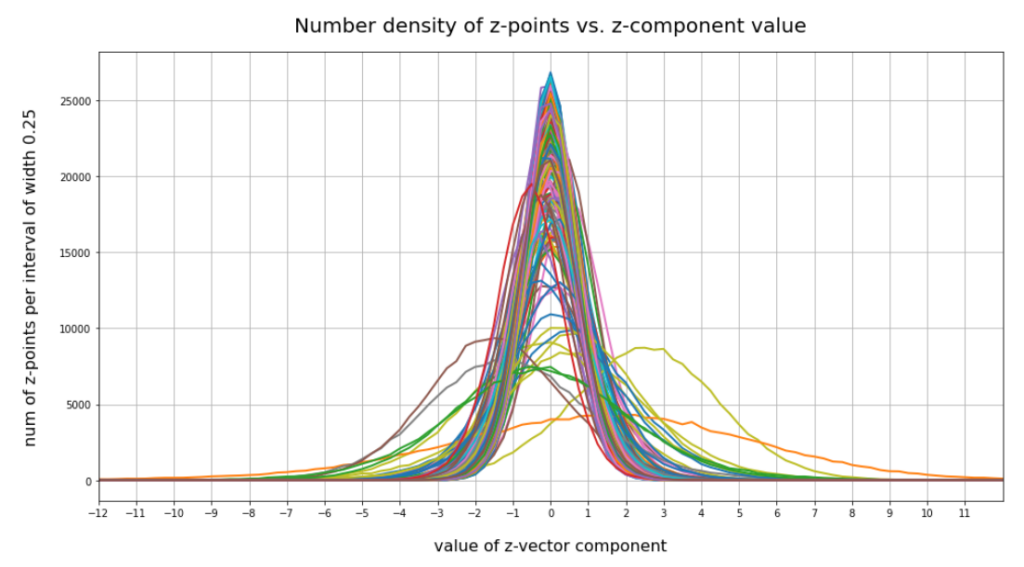Case II: Number distribution after 30 epochs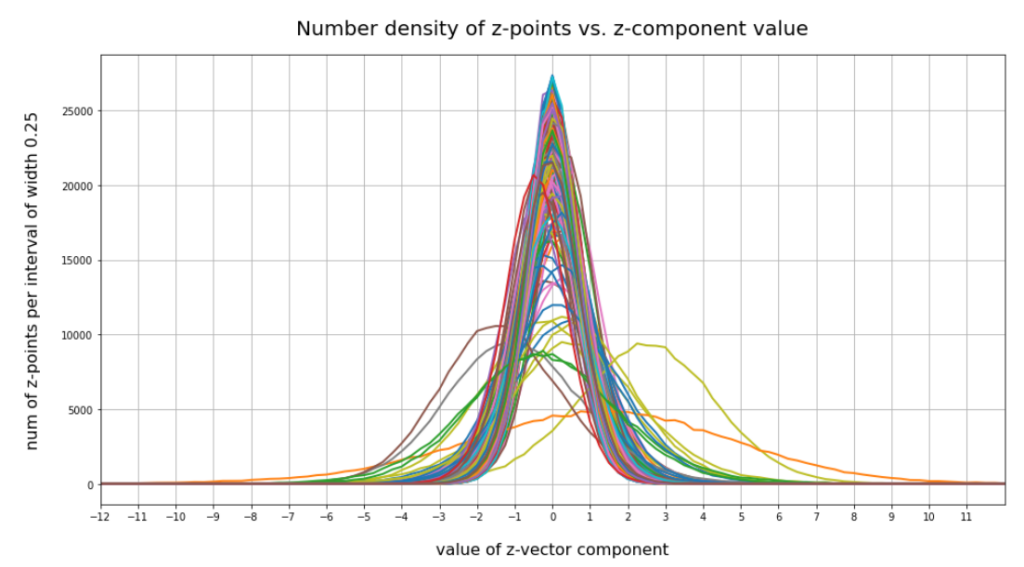We see that the individual number distributions are very similar to Gaussian distributions. For test case II I also give you the values for the central average value μ (named mu in the list below) and the half-width (named hw below) of the most interesting components. The half-width is the difference between those coordinate values where the distribution function achieves a value of half of the maximum number value at μ:

``` 15 mu : -0.25 :: hw:  1.5
16 mu :  0.5  :: hw:  1.125
56 mu :  0.0  :: hw:  1.625
58 mu :  0.25 :: hw:  2.125
66 mu :  0.25 :: hw:  1.5
68 mu :  0.0  :: hw:  2.0
110 mu :  0.5  :: hw:  1.875
118 mu :  2.25 :: hw:  2.25
151 mu :  1.5  :: hw:  4.125
177 mu : -1.0  :: hw:  2.25
178 mu :  0.5  :: hw:  1.875
180 mu : -0.25 :: hw:  1.5
188 mu :  0.25 :: hw:  1.75
195 mu : -1.5  :: hw:  2.0
202 mu : -0.5  :: hw:  2.25
204 mu : -0.5  :: hw:  1.25
210 mu :  0.0  :: hw:  1.75
230 mu :  0.25 :: hw:  1.5
242 mu : -0.25 :: hw:  2.375
253 mu : -0.5  :: hw:  1.0
```

These components obviously have either a relatively large absolute μ-value or a relatively large half-width. I call these components the dominant ones.

# Interpretation of the number distributions per vector component

The first thing these plots prove is the fact that the results for different networks are different, too. Without proving it, I also say from experience that even two different training runs for one and the same AE network structure may result in somewhat different number distributions. But although there are some differences there are also striking similarities:

1. Most of the components show a Gaussian like number distribution about some mean value.
2. The mean coordinate value μ for most of the components (≥ 90%) is zero or close to it.
3. The component values cover a region of [-12, 12] in our specific case.
4. There are only a few components ( ≤ 25) with mean values <μ> ≥ 0.25 or <μ> ≤ -0.25.
5. There are only a few components ( ≤ 10) with mean values <μ> ≥ +1.0 or <μ> ≤ -1.
6. There are only relatively few components (≤ 40) with a half-width ≥ 1.
7. There are only a few components (≤ 20) with a half-width ≥ 1.5
8. There are only very few components ≤ 5 with a half-width ≥ 3.

A bit of thinking and imagination tells us that the center of the distribution must be located somewhat off the origin, but very close to or within a hypervolume spanned by only a few dominant coordinate axes. The multi-dimensional region filled by the z-points has significant anisotropic extensions or elongations around its center only in a few directions of the multidimensional space.

All in all we speak about a very specific, limited multi-dimensional region, located at some distance from the origin (but not too far) with a center point close to or within a sub-volume of very low dimensions spanned by some coordinate axes. The overall direction of the center with respect to the origin is well defined by a few coordinates. The diameters of the regions are small in most directions. The z-points concentrate strongly towards the center of this region. Significant diameters around the center are only given in some particular directions. The respective axes involved are less than 10% of the total number.

This also means that a primary component analysis of the z-point distribution should only give us a number of dominant main components in the same number region (0.1 * N). See forthcoming posts for such an analysis.

Analogon in 3D: In an analogous case within a 3-dimensional space we would speak of a kind of ellipsoid with a significant diameter beyond 1 only in a specific direction and a center located close to a line in a plane spanned by 2 of the 3 coordinate axes.

# Comparison to the number distribution for statistically created vectors with a constant probability for component values in a specific interval

Now we look at the number distributions for all components of 200,000 vectors created by our method 2. We choose the same region for values [-12, 12] as displayed for our test cases I and II above. The following plot confirms what you certainly have already guessed: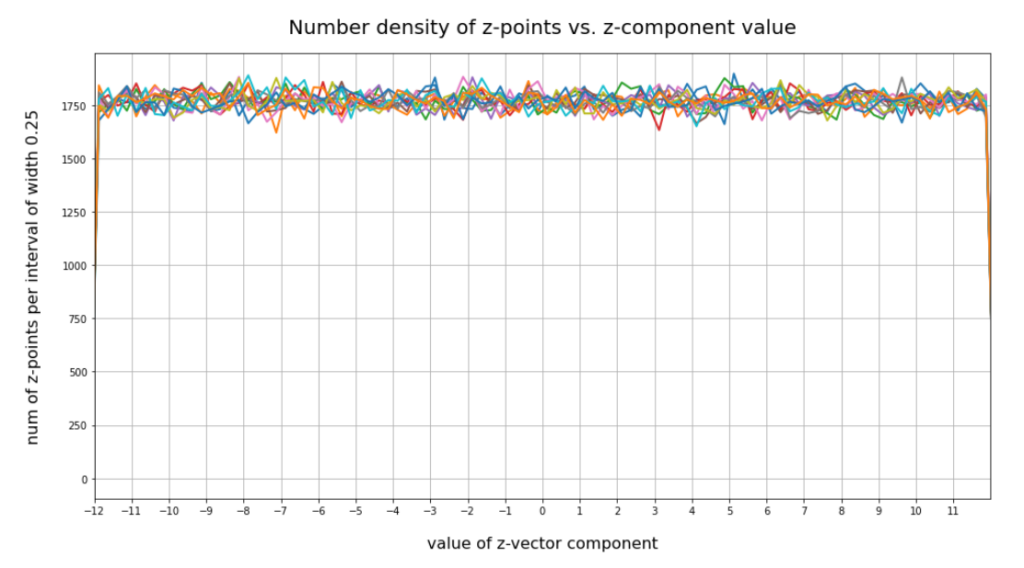This plot just reflects the design of our probability distribution for each of the components. But the plot also indicates clearly that most of our statistically created points will not hit the latent space region which is filled by the Autoencoder for CelebA data.

# The statistics obviously plays against us

It is very instructive to write a small program which scans all of the artificially created vectors and checks whether their end points lie within the region defined by the real CelebA points. I leave this to the reader. To get a good guess I personally defined a region of three times the half-width left and right of each component value center of the real distribution. The number of points fulfilling all criteria for our CelebA region came out to be exactly zero. Even with 12 times the half-width you get only very few vectors pointing into the CelebA region (around 20 vectors). Why is this the case?

# The curse of a high dimensionality and a constant probability distribution

The first point is that for some components of the CelebA vectors the half-width really is rather small. So you do not cover the whole value interval [-12, 12] for the component values, but only around 75% for 12 times the half-width. Let us assume that this is the case for 7 to 8 components. Let us further assume that another 50 components the width is 90%. Then the probability to get a point is (0.75)8 * 0.965 ≈ 0.1 * 0.0018 ≈ 0.00018. This gives us only 30 out of 170,000 vectors potentially fulfilling our conditions. We are in that range.

But we know already that 90% of all components should hit an interval [-3,3]. The probability for this in the case of our method 2 is 0.25 per component. The probability for a hit thus is 0.25220 which is zero for all practical purposes. This is it what really kills our efforts to place a point in the most interesting regions of the latent space with the help of method 2 and seemingly fitting values of b.

Now, you may think: A decisive parameter for method 2 ist the interval [-b, b] from which I pick my statistical component values. What if we diminish b? E.g. to b=3 or b=2? This is a good idea as we shall see in the next paragraph. Yet, you still get only a few vectors (< 10) fulfilling all criteria for two times the half-width.

Let us reduce our b-interval to [-3, 3] for vector creation. Then for a typical vector more than 50 components miss the target region. Test runs show that even for [-2, 2] only around 10 out of 170.000 vectors would hit (outer parts) of the target region for CelebA images. In this case the few components which have off-center mu values seemingly work against us.

Things change dramatically for b=1.5 and 2 times the half-width. Now the components of all the artificial vectors fulfill our criteria. If you, however, change the intervals to hit to 1.5 times the half-width you are back again to only a very few vectors (< 10). For b=1 and 1.5 times the half-width we get again a number of 170,000 vectors fulfilling our criteria.

Why does this happen? And does it mean that when we restrict our component values to [-1.5, 1.5] we would cover our real CelebA distribution?

# Number distributions for the vector lengths

Below you find a plot showing the number distribution with respect to typical vector lengths – both for CelebA (in red) and vectors artificially created with method 2 (other colors).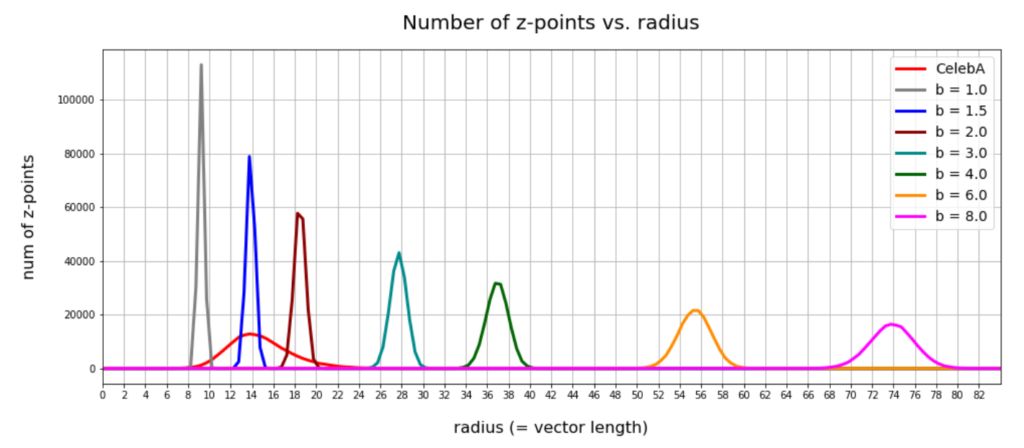The parameter “b” of our artificial distributions defines the interval [-b, b] from which we pick component values. The probability density is a constant for this interval.

The first point you may dwell upon is the fact that the radius values get so big – much bigger than b. This is due to the high number of dimensions; see the posts named for a mathematical cover of method 2.

The second counter-intuitive point may be the following: One expects that b=12 should really be a reasonable parameter value for method 2 to cover the range of component values for CelebA. But already for b=3 or b=4 we get vectors outside the vector length interval which CelebA latent vectors fill. The reason for this are properties of the artificial radius distributions which can be derived mathematically. A mathematical calculation of the expectation value for the mean length (= radius R) of our artificially generated vectors gives us a value around

<R>    ≈    b * sqrt(1/3 * N) * sqrt( 1 / (1 + 1/(4*N) )

with a relatively very narrow spread. See the derivation in the posts quoted above. sqrt in the formula above stands for the square root. The standard deviation Δstd(R) has a size of approximately

Δstd(R)    ≈    b * sqrt(1/15 * N) * sqrt( 1 + 1/(4*N) )

The ratio of the half-width to radius thus declines with the square root of the number of dimensions N. As we see, only the artificial distributions for b=1, b=1.5, b=2 cover parts of the radius distribution for latent CelebA vectors.

So, if we want to use method 2 then we should work with b-values 1.0 ≤ b ≤ 2.0 to get a probability > 0 for creating reasonable images of human faces.

# Will a proper value of b guarantee us reasonable face images?

We have seen that b must be reduced to a range 1.0 ≤ b ≤ 2.0 to get reasonable radius values of our statistical vectors. Unfortunately, this does not guarantee us proper images either. The reason is that there might be correlations between the dominant component values which our simple number distributions do not reveal. We will take care of this in the next post. For now I just show you a plot of the correlation between two specific dominant components for 1000 randomly selected CelebA latent vectors: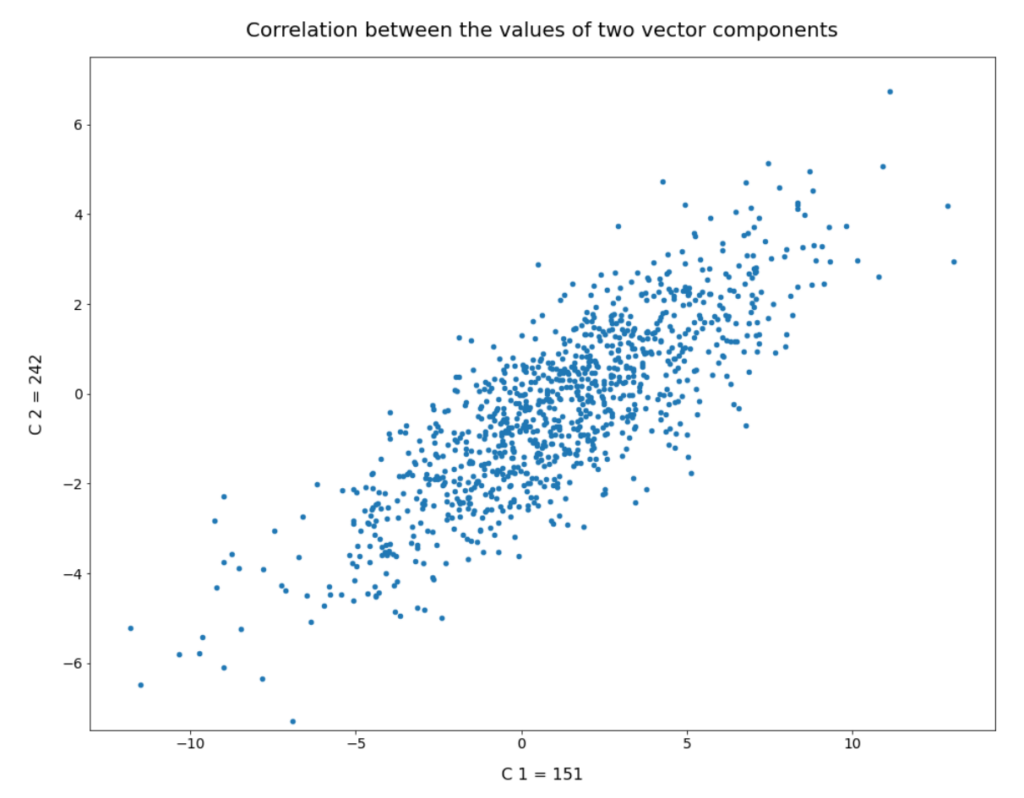# Conclusion

In this post we have partially analyzed the distribution of vectors and related z-points which an Autoencoder creates for CelebA images in its latent space. We have found that the number distributions per vector component look like Gaussian distributions. While most of the components have a small spread around the value zero, there are a few dominant components which determine the (off-center) location and orientation of a coherent, confined and ellipsoidally shaped region for CelebA z-points. The center of this region is close to a hypervolume defined by a few axes.

It was a bit counter-intuitive to see that a simple method to create statistical z-points via a constant probability distribution for individual component values would obviously miss the relevant latent region for CelebA images totally. We saw that we would need very special parameter values to limit the component values to get artificial latent vectors with the required length. These findings alone make it very improbable that arbitrary z-points created without some restrictions for their component values would lead to reasonable face image creation by the Decoder.

Something that we have not yet covered is the question of correlations between vector components. This is the topic of the next post: